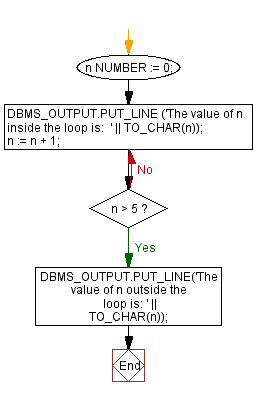﻿ PL/SQL Control Statement: Print the value of a variable inside and outside a loop using LOOP EXIT statement - w3resource# PL/SQL Control Statement Exercises: Print the value of a variable inside and outside a loop using LOOP EXIT statement

## PL/SQL Control Statement: Exercise-16 with Solution

Write a program in PL/SQL to print the value of a variable inside and outside a loop using LOOP EXIT statement.

Sample Solution:

PL/SQL Code:

``````DECLARE
n NUMBER := 0;
BEGIN
LOOP
DBMS_OUTPUT.PUT_LINE ('The value of n inside the loop is:  ' || TO_CHAR(n));
n := n + 1;
IF n > 5 THEN
EXIT;
END IF;
END LOOP;
DBMS_OUTPUT.PUT_LINE('The value of n outside the loop is: ' || TO_CHAR(n));
END;
/
```
```

Flowchart:Sample Output:

```SQL> /
The value of n inside the loop is:  0
The value of n inside the loop is:  1
The value of n inside the loop is:  2
The value of n inside the loop is:  3
The value of n inside the loop is:  4
The value of n inside the loop is:  5
The value of n outside the loop is: 6

PL/SQL procedure successfully completed.
```

Improve this sample solution and post your code through Disqus

What is the difficulty level of this exercise?

﻿